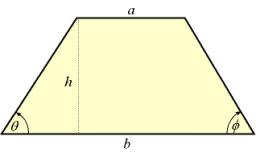# Trapezoid 65644

In an isosceles trapezoid, the base ratio a / c = 9/7, arm b = 10 cm, height v = 8 cm. Calculate the area of the trapezoid in cm².

S =  384 cm2

### Step-by-step explanation:Did you find an error or inaccuracy? Feel free to write us. Thank you!

Tips for related online calculators
Need help calculating sum, simplifying, or multiplying fractions? Try our fraction calculator.
Check out our ratio calculator.
Do you have a linear equation or system of equations and looking for its solution? Or do you have a quadratic equation?
The Pythagorean theorem is the base for the right triangle calculator.